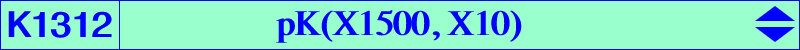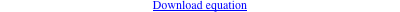too complicated to be written here. Click on the link to download a text file.X(10), X(37), X(42), X(65), X(71), X(210), X(227), X(1826), X(53011), X(53012), X(53013) Geometric properties :K366 is the locus of pseudo-pivots of central psKs with center X(10). The locus of pseudo-poles is K1311 = pK(X762, X10) and the locus of pseudo-isopivots is K1312 = pK(X1500, X10). One of these cubics is K033 and all four cubics are equivalent to K002. See Table 21. K1312 is the barycentric products X(10) × K002, X(37) × K034, X(42) × K184, X(71) × K647, X(306) × K445, X(313) × K346, X(321) × K175, X(1826) × K099, also the barycentric quotient K576 ÷ X(1790). K1047 is the image of K1312 under the symbolic substitution SS{a → SA}.